/ KITCTFCTF  REV

# protector

This was a cool reversing challenge where I wrote a GDB script to undo obfuscated operations to get the flag.

## Setup

We’re given a binary called `protector`, and if we run it, we’re greeted with an input prompt.

``````Input:
``````

If we enter anything random, we just get hit with a:

``````No
``````

If we open the binary in Ghidra, we see that this writes some code to an address and immediately jumps to it, indicating self-modifying code.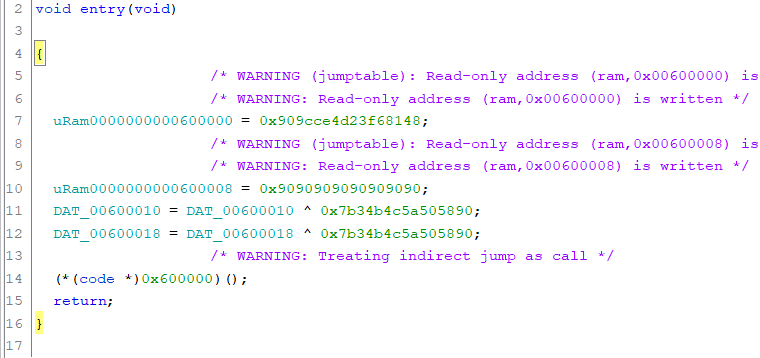Let’s open the binary in GDB and see what’s going on.

## GDB Exploration

First, I wanted to see what was happening around the read syscall. I ran the program with the `r` command and then interrupted the program using `ctrl+c` when it was waiting for user input. I printed out the instructions around that area using `x/17i \$rip-2`: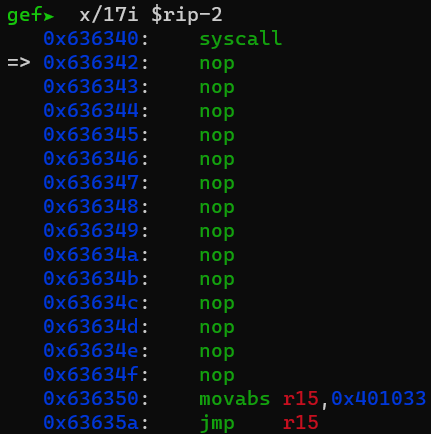It appears that the code is obfuscated so that it executes a couple of instructions before jumping elsewhere. I stepped through more instructions, but it didn’t seem simple enough to know what was going on immediately.

I was stuck for a bit, but then I got the idea to put a read watchpoint on the string storing the input. The register view from gef showed me that the input was stored in `\$rsp`, and I used the `rwatch` command to set a read watchpoint on that address.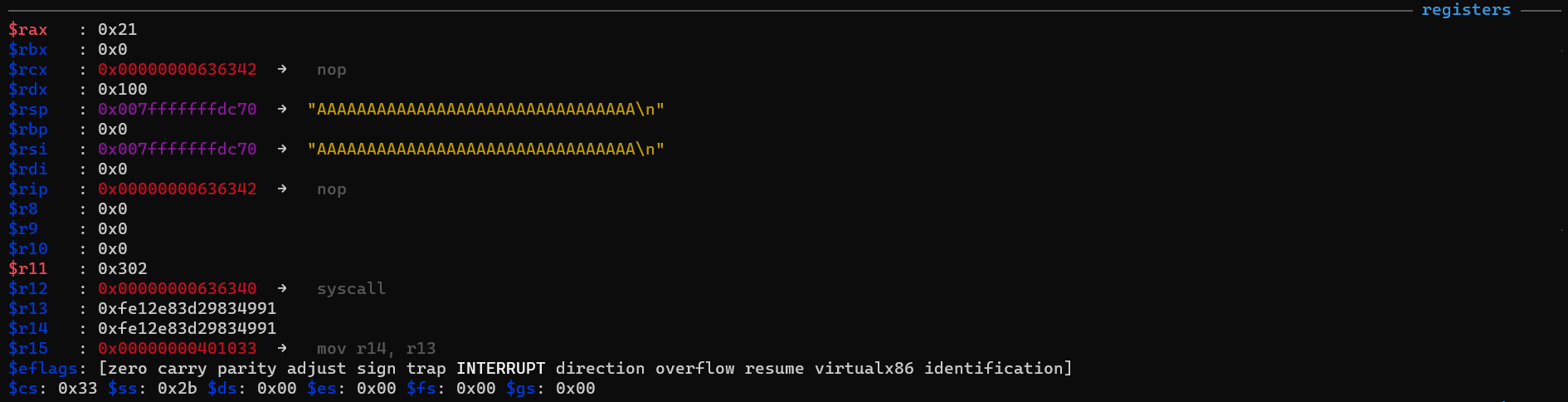The watchpoint was hit! I looked around the area to figure out which instruction caused the read, and it was a `CMP` instruction.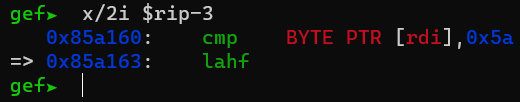Using the stack view from gef, I was able to see that my input had completely changed.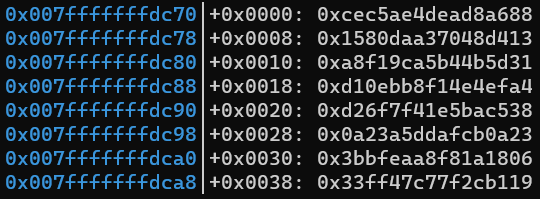From these observations, I concluded that the input had undergone some transformation, and that the first character needed to be `0x5a` after the transformation. To determine what transformations were done to the input, I set a write watchpoint on the input using the `watch` command. I restarted the program and noticed that each character only had three different instructions that modified it: `XOR`, `ADD`, and `SUB`.

These operations are all reversible, so if we can record all the operations that are done to each character and the expected final value, we can compute the flag.

There were too many characters to do this manually, so I learned how to use the Python GDB API and wrote a script.

### GDB Script

Let’s go over the code. First, I defined some useful constants.

``````FLAG_START = 0
FLAG_SIZE = 64
WORD_SIZE = 4

ops = []
for _ in range(FLAG_SIZE):
ops.append([])
expected = []
``````

`WORD_SIZE` defines how many bytes each watchpoint will watch. This happened to be 4 on my system. `FLAG_START` and `FLAG_SIZE` define the range of the input that has the flag. I determined `FLAG_SIZE` incrementally, starting from 0 and going up until I got the entire flag. `ops` is a list containing lists of operations that are done to each character (the index of `ops` corresponds to the index in the flag). `expected` contains the expected final value of each character.

This code appears in the `SolveChallenge` class:

``````class SolveChallenge(gdb.Command):
def __init__(self):
super(SolveChallenge, self).__init__("solve", gdb.COMMAND_DATA)
``````

This defines a class that binds itself to the `solve` keyword. After sourcing the script using the `source` command, executing the `solve` command will run the invoke function of the script:

``````def invoke(self, arg, from_tty):
for i in range(FLAG_START, FLAG_SIZE, WORD_SIZE):
gdb.execute("del")
gdb.execute("r < input.txt")
gdb.execute("c")

while True:
gdb.execute("c")
instr = get_instr()
# Calculate the inverse op
op = None
match instr:
case "xor":
op = operator.xor
op = operator.sub
case "sub":
case "cmp":
break
case _:
raise Exception(f"Unknown op: {instr}")
assert instr == "BYTE" and instr == "PTR"

operand = instr[-1].split(",")
assert operand == "[rdi]"
operand = int(operand, 16)
ops[flag_i].append((op, operand))

for i in range(FLAG_START, FLAG_SIZE, WORD_SIZE):
gdb.execute("del")
gdb.execute("r < input.txt")
gdb.execute("c")
for i in range(FLAG_SIZE):
gdb.execute("c")
break
instr = get_instr()
while instr != "cmp":
assert instr in ["xor", "add", "sub"]
gdb.execute("c")
instr = get_instr()
continue
assert instr[:3] == ["cmp", "BYTE", "PTR"]
operand = instr[-1].split(",")
assert operand == "[rdi]"
expected.append(int(operand, 16))
print("Checked", len(expected))
calc_flag()
``````

This is a long function, but let’s break it down. It consists of two loops. The first loop calculates the `ops` list, and the second loop calculates the `expected` list.

Both loops iterate through all the characters of the flag using watchpoints. Since each watchpoint corresponds to 4 bytes, each iteration corresponds to 4 characters. Both loops also start with the following code:

``````gdb.execute("del")
gdb.execute("r < input.txt")
gdb.execute("c")
``````

This deletes any existing watchpoints and sets a breakpoint on the read syscall. The `catch` command breaks twice for every syscall: once before it’s run, and once after. The `c` command tells gdb to continue execution after the syscall is run.

We also need to compute `start_adddr`, which keeps track of the address of the input. It’s set exactly once by reading `rsp`, using the following code:

``````if start_addr is None:
``````

The rest of the code in the first loop continuously breaks whenever the write watchpoint is hit and records the inverse operation that was done to the character. We stop once we hit the `CMP` instruction from the read watchpoint.

The rest of the code in the second loop waits until the `CMP` instruction and records the expected final value of the character.

Finally, the `calc_flag` function computes the flag:

``````def calc_flag():
for c_ops, c_expected in zip(ops, expected):
val = c_expected
for op, operand in reversed(c_ops):
val = op(val, operand) & 0xFF
print(chr(val), end="")
print()
``````

Here’s the full code:

``````import gdb
import operator

FLAG_START = 0
FLAG_SIZE = 64
WORD_SIZE = 4

ops = []
for _ in range(FLAG_SIZE):
ops.append([])
expected = []

def calc_flag():
for c_ops, c_expected in zip(ops, expected):
val = c_expected
for op, operand in reversed(c_ops):
val = op(val, operand) & 0xFF
print(chr(val), end="")
print()

def get_instr():
return gdb.execute("x/i \$rip-3", to_string=True).split("\t")[-1].strip().split()

class SolveChallenge(gdb.Command):
def __init__(self):
super(SolveChallenge, self).__init__("solve", gdb.COMMAND_DATA)

def invoke(self, arg, from_tty):
for i in range(FLAG_START, FLAG_SIZE, WORD_SIZE):
gdb.execute("del")
gdb.execute("r < input.txt")
gdb.execute("c")

while True:
gdb.execute("c")
instr = get_instr()
# Calculate the inverse op
op = None
match instr:
case "xor":
op = operator.xor
op = operator.sub
case "sub":
case "cmp":
break
case _:
raise Exception(f"Unknown op: {instr}")
assert instr == "BYTE" and instr == "PTR"

operand = instr[-1].split(",")
assert operand == "[rdi]"
operand = int(operand, 16)
ops[flag_i].append((op, operand))

for i in range(FLAG_START, FLAG_SIZE, WORD_SIZE):
gdb.execute("del")
gdb.execute("r < input.txt")
gdb.execute("c")
for i in range(FLAG_SIZE):
gdb.execute("c")
break
instr = get_instr()
while instr != "cmp":
assert instr in ["xor", "add", "sub"]
gdb.execute("c")
instr = get_instr()
continue
assert instr[:3] == ["cmp", "BYTE", "PTR"]
operand = instr[-1].split(",")
assert operand == "[rdi]"